Next: Measurement Error Matrix at Up: No Title Previous: Summary

# Effects of Thin Layer Multiple Scattering

Consider a particle passing through a thin layer, whose track parameter vector changes from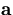to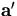due to multiple scattering there. We assume here that the track parameter vector of the particle is measured after the multiple scattering to be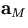with its error matrix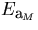. In the Gaussian approximation valid for small angle multiple scattering, the probability of gettingwhen the true track parameter vector before the multiple scattering isis given by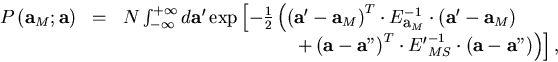(1)
where E'MS is the diagonalized error matrix corresponding to multiple scatteringN is a normalization factor depending only onand E'MS, and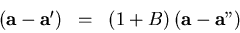with B being a matrix whose only nonzero component is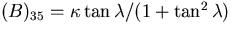.Making use of the fact that B is nilpotent, i.e. B2 = 0, we can easily eliminate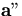from the exponent of Eq.A.1: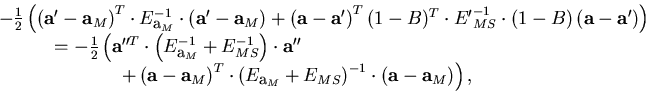(3)
where we have defined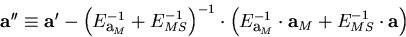(4)
and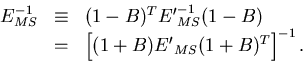(5)
Changing the integration variable fromto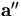, Eq.A.1 now becomes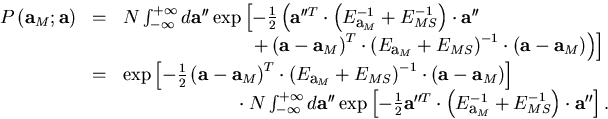(6)
Notice that the last line is a constant independent ofand,which proves that the error matrix including the multiple scattering has to be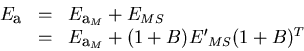(7)

The derivation of the explicit form of E'MS is straightforward. The multiple scattering changes the helix parameter vector fromtoand, when the pivot is chosen to be the point of the multiple scattering, the tangential vector thereat changes as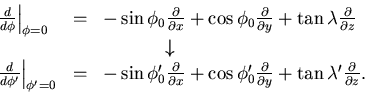(8)
Then the direction change of the track in space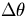is given by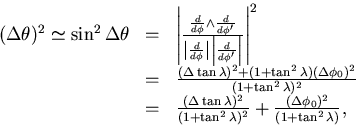(9)
where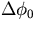and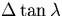are defined as components of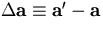.The above equation determines E'MS through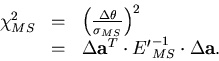(10)Next: Measurement Error Matrix at Up: No Title Previous: Summary
Keisuke Fujii
12/4/1998